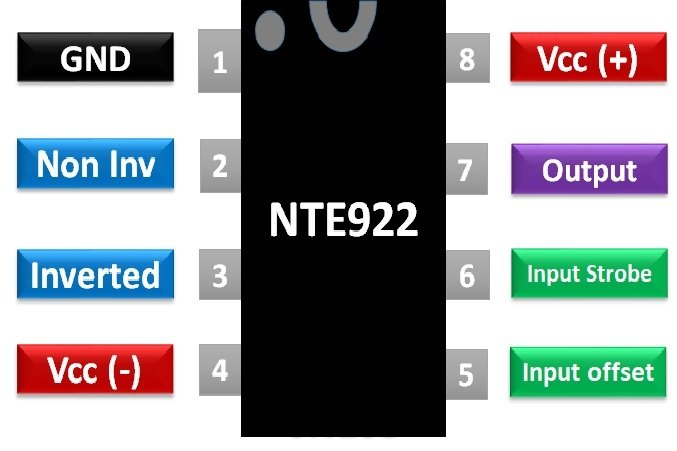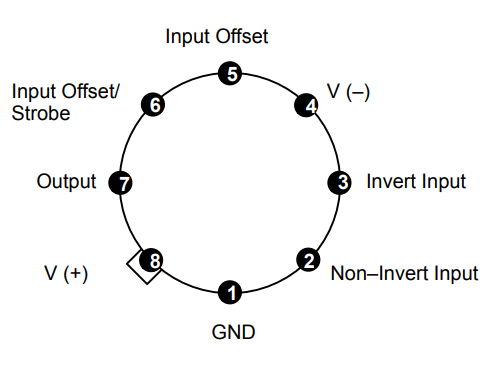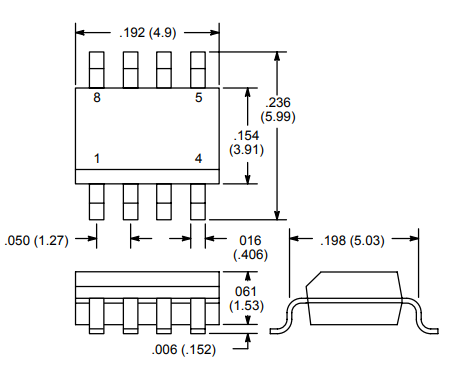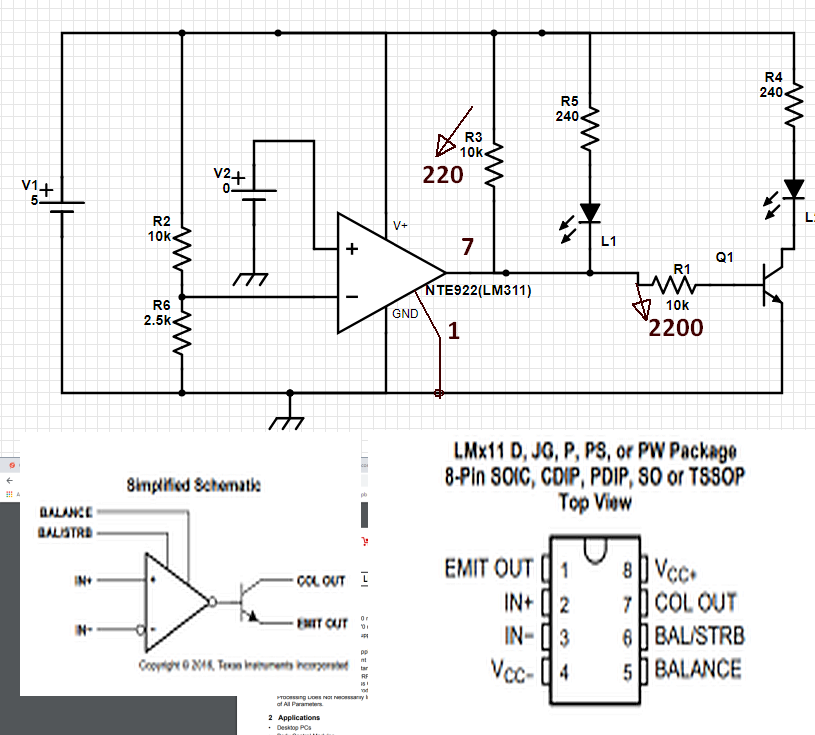# NTE922 Voltage Comparator IC Pinout and Example

Voltage comparator IC NTE922 is basically a comparator which is responsible for comparing two levels of voltages. It is available as NTE922, NTE922M, and NTE922SM. NTE922 is an eight pin metal can package, NTE922M is an eight pin Mini DIP package and NTE922SM is eight pin SOIC surface mount package. NTE922 packages have the advantage of having low input current. This current is around a thousand times less than NTE 910 packages. For IC logic NTE922 can operate on a wide range of supply voltages. These voltages vary from 5V to ±15V.

This device is less affected by stray oscillations. Its output is quite consonant with RTL, TTL, DTL, and MOS. Lamps and relays can easily be operated by using NTE922 with a high value of current as 50mA and 50V switching voltages. The isolation of input and output from the common ground is possible with output measured with reference to ground. The voltage comparator operates from a single supply voltage of 5V. The input current and offset current is 150nA and 20nA respectively. The differential input voltage range is ±30V while the power consumption is 135mW at a voltage of ±15V.

## NTE922 Pinout Diagram

NTE922 comes as 8 PIN IC with basic pins for applying inverting and non-inverting inputs and for obtaining the output. The pin configuration diagram is given below:Details and functionality of each pin for this voltage comparator IC are listed in the table.

PINS Details
1 ( Ground) This pin is for applying ground
2 ( Non-Inverting Input) This pin for applying non-inverting input voltage
3 (Inverting Input) This pin is for applying inverting input voltage
4 (-Vcc) This pin is for applying negative DC supply
5 (Input Offset) This pin is for adjusting offset
6 (Input Offset/ Strobe) This pin is for adjusting offset
7 (Output) This pin is for obtaining output
8 (+Vcc) This pin is for applying positive DC supply

The pin connection diagram for NTE922 8-pin metal can package is shown as:### 2D dimension diagram

The physical dimensions of voltage comparator are as follow:## NTE922 Voltage Comparator Features

 Parameters NTE922 Input offset voltage (mV) 7.5 Input bias current (nA) 250 Strobe On current (mA) 3 Saturation voltage (V) 1.5 Voltage gain (V/mV) 200 Saturation Voltage (V) 1.5 Response time (ns) 200 Input offset current (nA) 50 Output leakage current (nA) 59 Output short circuit duration (s) 10 Operating temperature range (°C) 0 to 70 Supply Voltage (V) ±15 Power dissipation (mW) 135 Differential Input Voltage (V) ±30 Lead temperature (Soldering, 10s) (°C) 260 Voltage at strobe pin (V) V+ -5

## Where and How to Use NTE922

NTE922 can be used by applying +Vcc as 5V and –Vcc as 0V. Since it is a comparator IC, so if input at pin no. 2 is greater than input at pin no. 3 i.e. non-inverting input voltage is greater than inverting voltage the output will be high or in other words pin no. 7 will be at high potential. If it is required to adjust the DC offset voltage, pin no. 5 and 6 are used. These pins must be short circuited if they are not in use. Strobe pin should not be short circuited to the ground. It should be run by the current 3 to 5mA.

NTE922 can be used for the construction of:

• Null detectors
• Zero-crossing detectors
• Relaxation oscillator
• Level shifter
• Analog to digital convertor
• Window detector
• Absolute value detector

One of the common use of NTE922 is the null detector. These detectors compare the unknown voltage with the reference voltage. The reference voltage is applied as non-inverting input while unknown as inverting input. If the two voltages are of the same value, null detection will be achieved. This application makes NTE922 eligible for digital sensing and can be employed in various sensing circuits.

## NTE922 Examples

This circuit diagram is an example of an alternate LED flasher based on voltage comparator. This voltage comparator circuit turns on one LED when the voltage is above -1V and turns up another LED when the voltage is below this level. This is a simplest example to use this voltage comparator IC.## Applications

The real-life applications of NTE922 are:

• Oscillator circuits
• Comparator circuits
• Sensor circuits
• Window detector
• A-D converter